# 4. Find the product(s) of the following reaction: 1. CH2MgBr, excess CI 2. H30+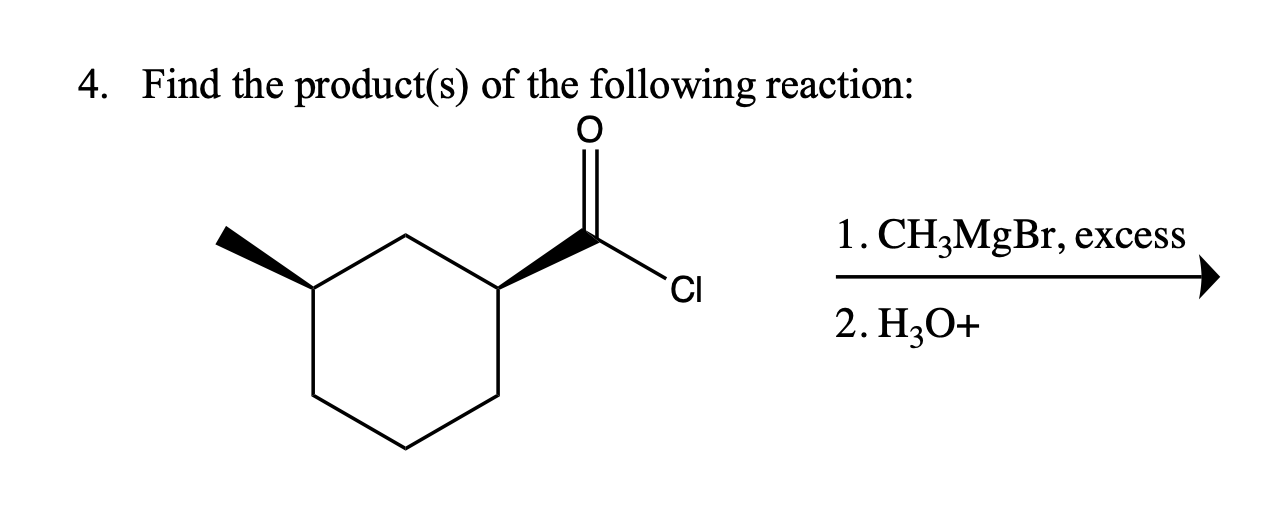4. Find the product(s) of the following reaction: 1. CH2MgBr, excess CI 2. H30+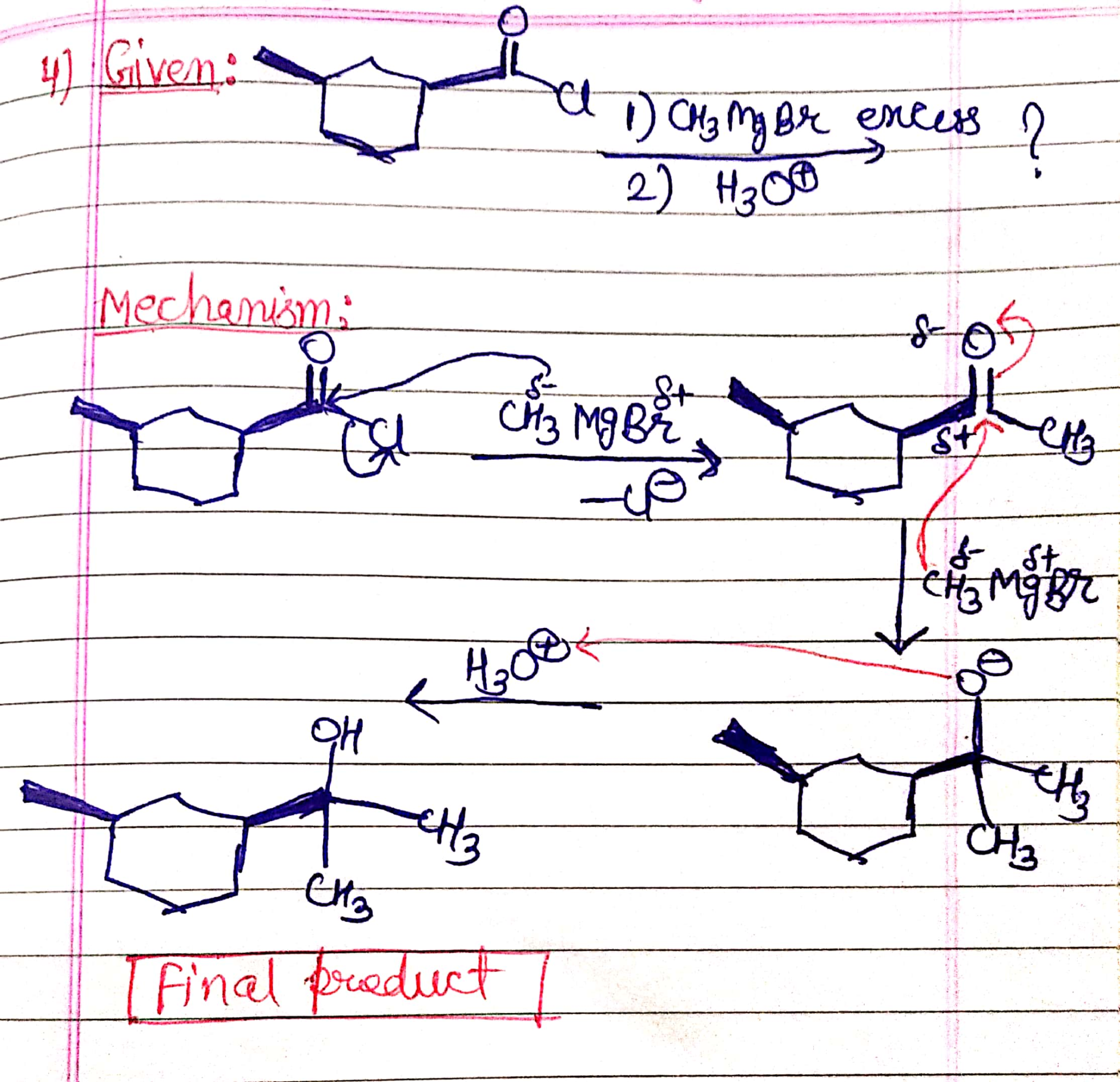##### Add Answer of: 4. Find the product(s) of the following reaction: 1. CH2MgBr, excess CI 2. H30+
Similar Homework Help Questions
• ### 4. Find the product(s) of the following reaction: o 1. CH_MgBr, excess CI 2. H30+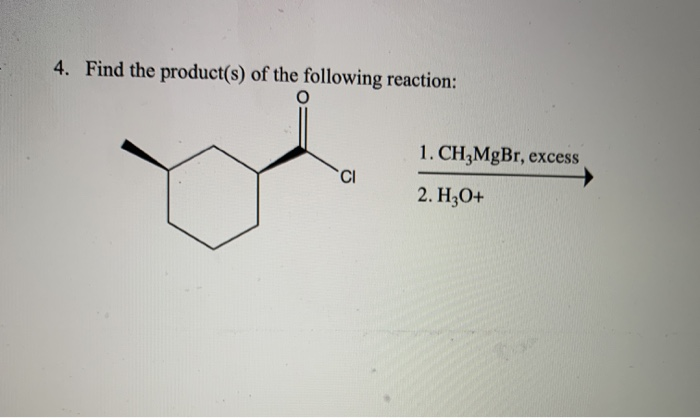4. Find the product(s) of the following reaction: o 1. CH_MgBr, excess CI 2. H30+

• ### 4. Find the product(s) of the following reaction: 1. CH_MgBr, excess CI 2. H3O+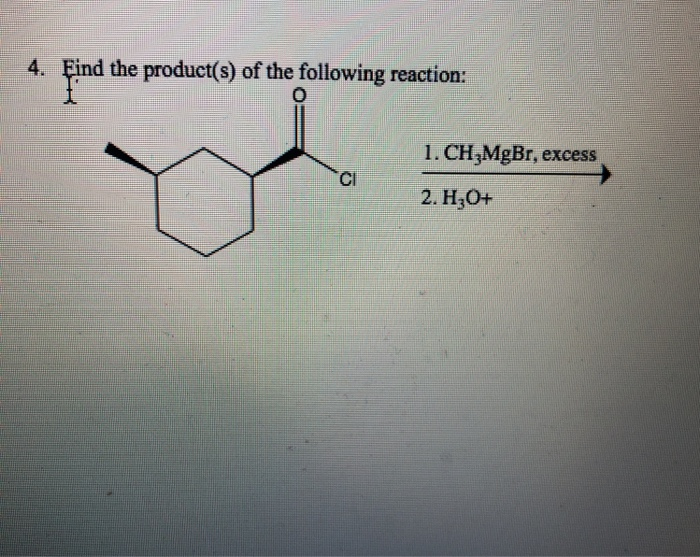4. Find the product(s) of the following reaction: 1. CH_MgBr, excess CI 2. H3O+

• ### 4. Find the product(s) of the following reaction: o CH3MgBr, excess CI 2. H3O+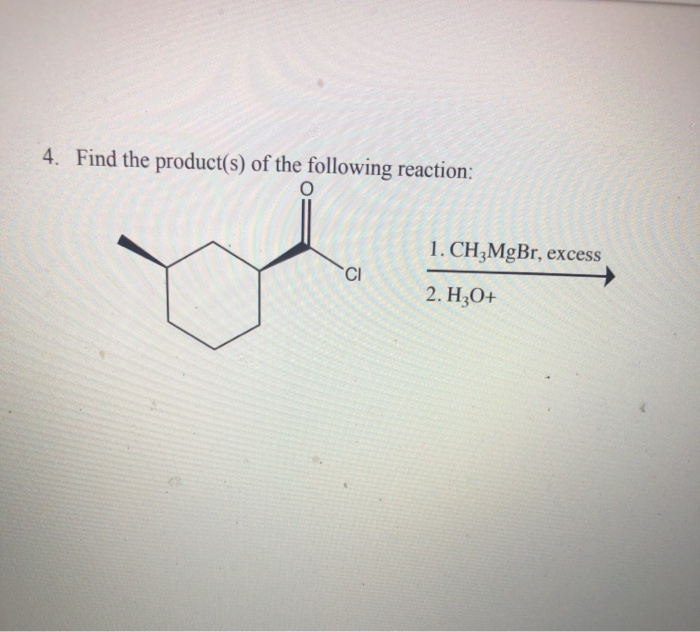4. Find the product(s) of the following reaction: o CH3MgBr, excess CI 2. H3O+

• ### 4. Find the product(s) of the following reaction: CI 1. CH MgBr, excess 2. H2O+ 5....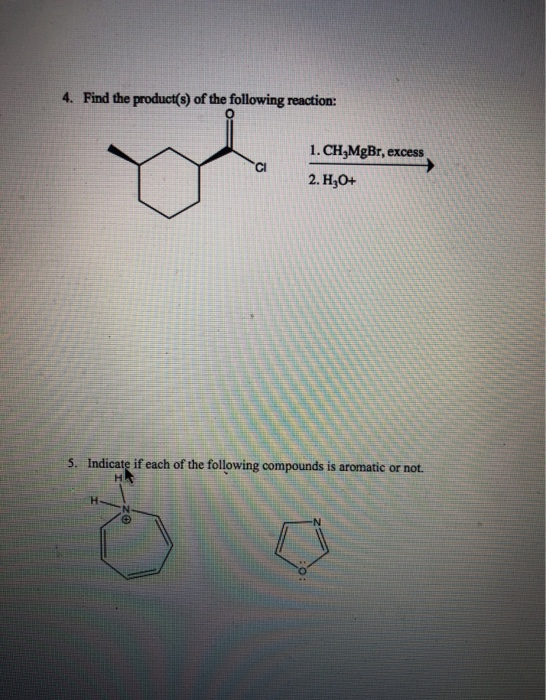4. Find the product(s) of the following reaction: CI 1. CH MgBr, excess 2. H2O+ 5. Indicate if each of the following compounds is aromatic or not. H

• ### 4. Find the product(s) of the following reaction: o 1. CH3MgBr, excess CI 2. H2O+ 5....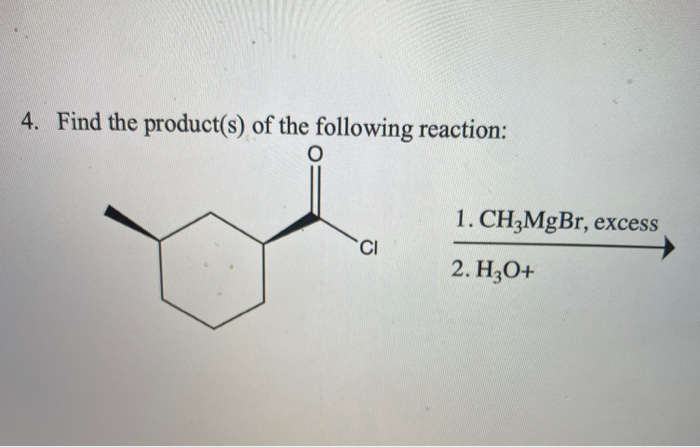4. Find the product(s) of the following reaction: o 1. CH3MgBr, excess CI 2. H2O+ 5. Indicate if each of the following compounds is aromatic or not. H H Zo -N 10:

• ### 4. Find the product(s) of the following reaction: 1. CH,MgBr, excess CI 2. Hz0+ I 5....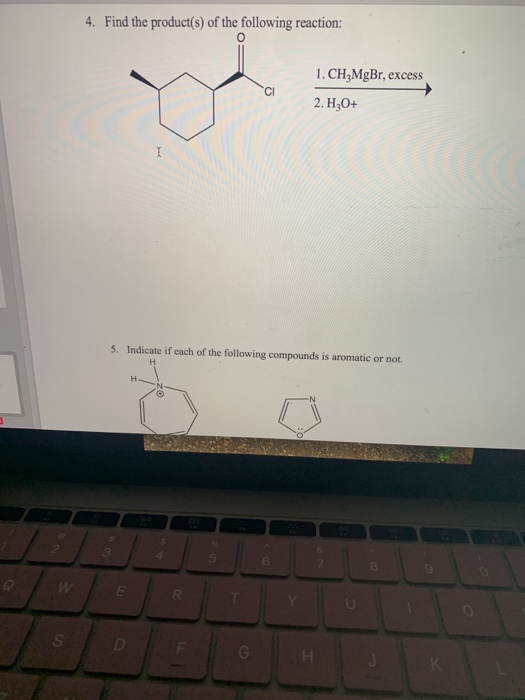4. Find the product(s) of the following reaction: 1. CH,MgBr, excess CI 2. Hz0+ I 5. Indicate if each of the following compounds is aromatic or not. H 3 5 6 8 W IR S D G Н.

• ### 4. Find the product(s) of the following reaction: o 1. CH3MgBr, excess 2. H2O+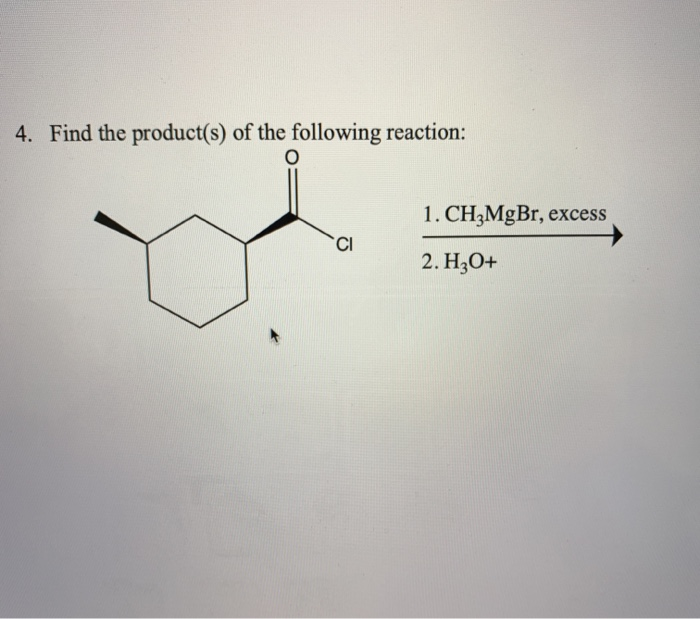4. Find the product(s) of the following reaction: o 1. CH3MgBr, excess 2. H2O+

• ### 10. Find the product(s) of the following reaction: 1. NaOC2H5 + 2. H30* 3. Ethanol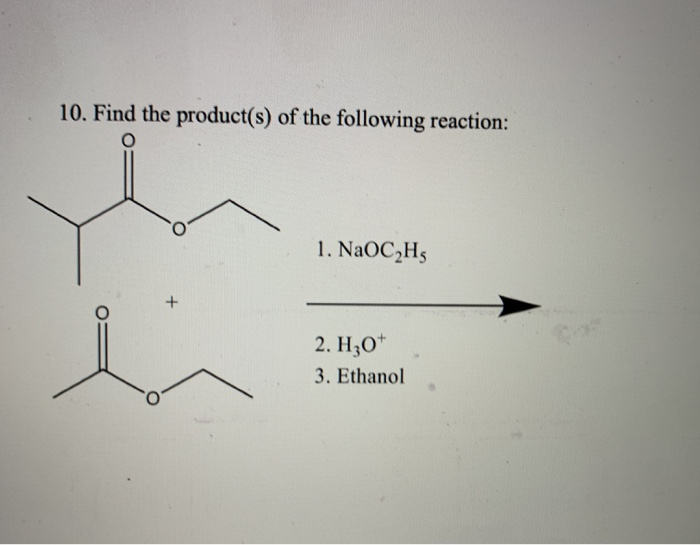10. Find the product(s) of the following reaction: 1. NaOC2H5 + 2. H30* 3. Ethanol

• ### 10. Find the product(s) of the following reaction: 1. NaOCH + 2. H30+ 3. Ethanol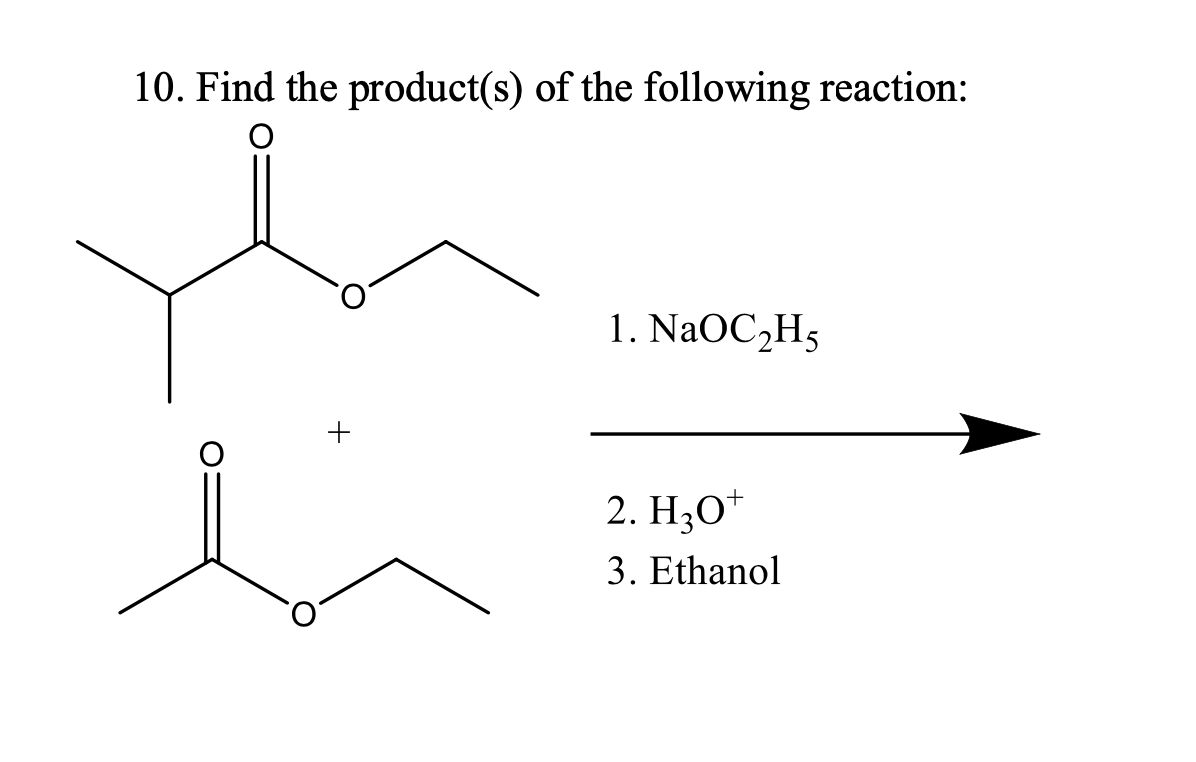10. Find the product(s) of the following reaction: 1. NaOCH + 2. H30+ 3. Ethanol

• ### 4. Find the product(s) of the following reaction: 1. CH,MgBr, excess 2. H,O+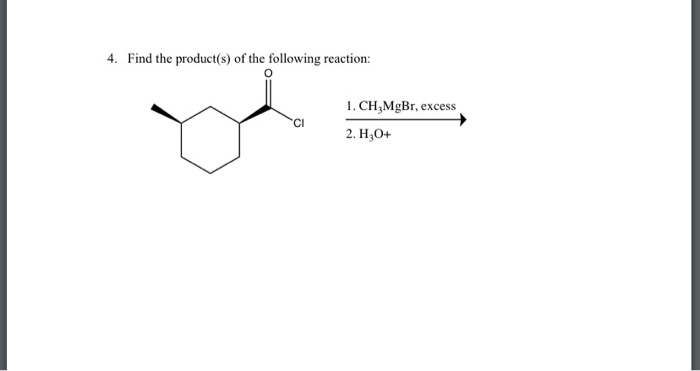4. Find the product(s) of the following reaction: 1. CH,MgBr, excess 2. H,O+

Free Homework App